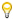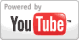Calculating the Probability of Simple Events
Grade: 9 - 12 | The lesson explains the basic idea of probability, and a few simple examples of calculating the probability of simple events.Add to My DashboardProbability
For Other Topics :Quiz Games by TopicInteractive DiagramsFlow Chart GamesVocabulary GamesSlide PuzzlesJigsaw PuzzlesCreate PresentationsBrain GamesVideos by Topic### Search Videos & Games